# Threephase AC circuits

## Electricity and Electronics

• #### Question 1

Suppose you need to design a three-phase electric heater to dissipate 15 kW of heat when powered by 480 VAC. Your options are to build a delta-connected heater array or a wye-connected heater array:Calculate the proper resistance value for each array, to achieve the desired heat output:

$R_{delta}$ =

$R_{wye}$ =

Reveal answer
• #### Question 2

A three-phase electric motor operating at a line voltage of 4160 volts AC (RMS) draws 27.5 amps of current (RMS) through each of its lines. Calculate the amount of apparent power consumed by this motor.

Assuming the motor is 92

Reveal answer
• #### Question 3

Suppose the current through each of the ammeters is 2.81 amps, and the ratio of each current transformer is 100:5. Calculate the horsepower output of this AC motor, assuming a power factor of 1 and an efficiency of 88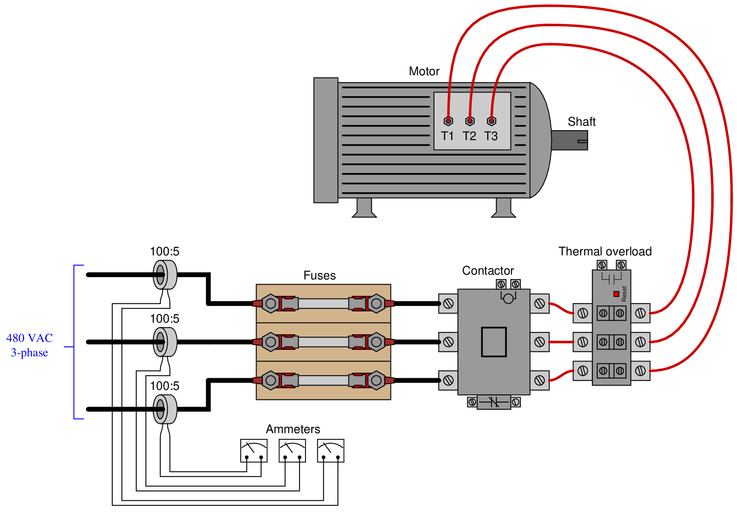$P$ =

Reveal answer
• #### Question 4

Examine the primary and secondary connections on this three-phase transformer bank, and then determine the line voltage to the customer, assuming 12.5 kV line voltage on the distribution power lines. The schematic diagram shown in the grey box is typical for each of the three transformers: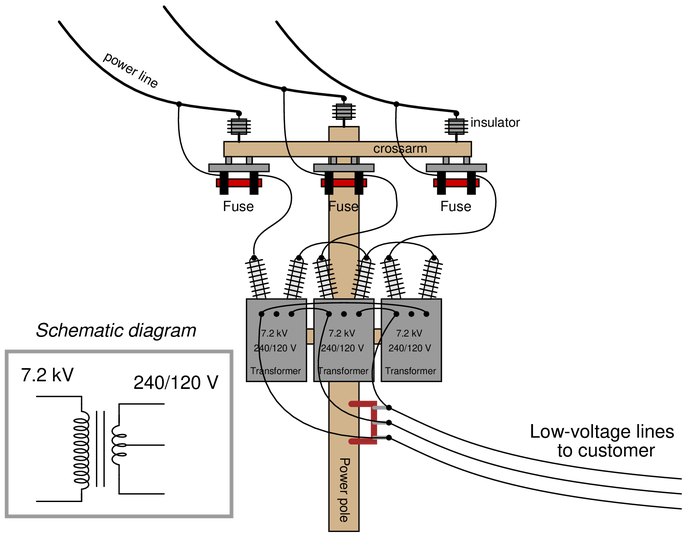Reveal answer
• #### Question 5

Three step-down transformers have their primary (high-voltage) terminals connected together in a wye’’ condiguration so that the 12.5 kV line voltage energizes each primary winding with 7.2 kV. The secondary terminals on each transformer have been left disconnected: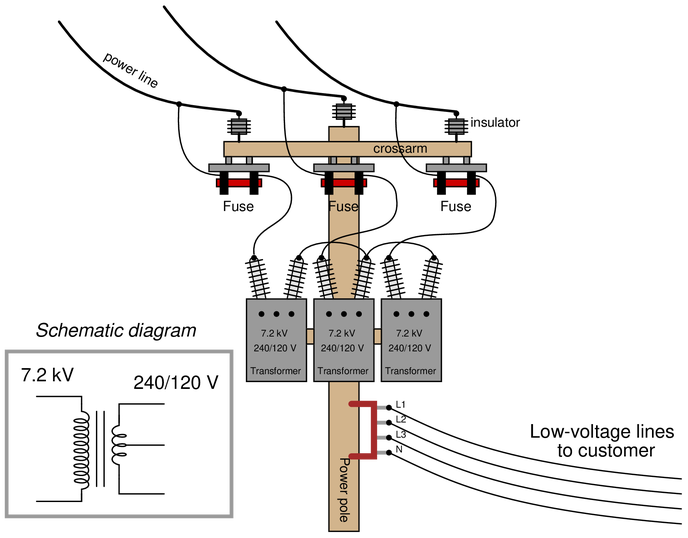Sketch proper wire connections to provide 120/208 VAC to the customer.

Reveal answer
• #### Question 6

Calculate the operating current through each of the load resistances shown in this circuit (assuming each three-phase load is balanced):Also, calculate the power dissipated by each load.

Reveal answer
• #### Question 7

A 15 kV three-phase alternator needs to have its windings connected properly to prepare it to send power to a bus’’ shared by other alternators in a power plant: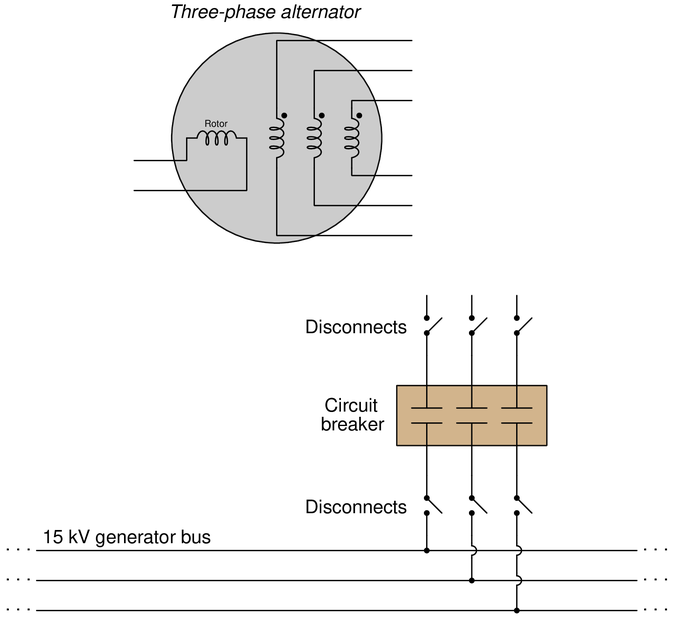Each phase winding on the alternator is rated at 15 kV. The rotor winding is rated at 220 VDC. Sketch all necessary connections to make this alternator work as intended.

Reveal answer
• #### Question 8

An {\it unbalanced} wye-connected load receives power from a balanced 120/208 VAC source: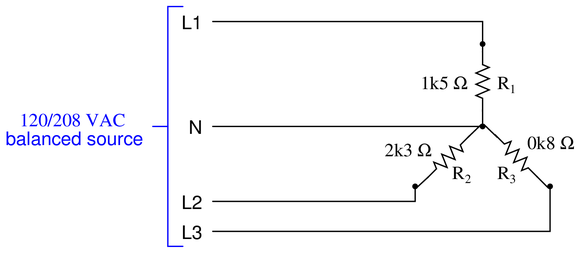Calculate the current through each of the three lines (L1, L2, and L3), as well as the current through the neutral conductor:

{\bullet} $I_{L1}$ =

{\bullet} $I_{L2}$ =

{\bullet} $I_{L3}$ =

{\bullet} $I_{N}$ =

Reveal answer
• #### Question 9

A three-phase step-down transformer supplies 480 VAC to a pair of resistive loads. The secondary winding is corner-grounded’’ on the X2 leg: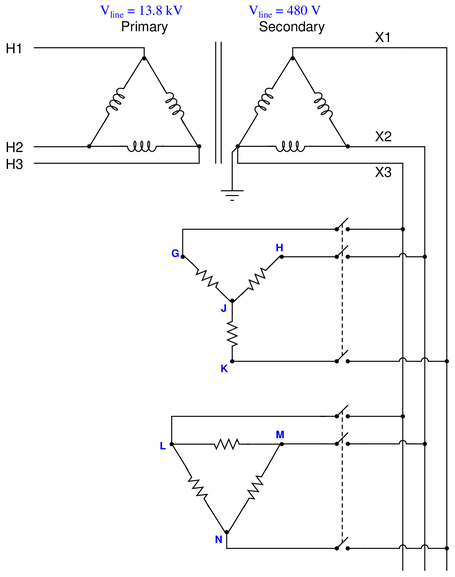Determine the following phase-to-ground voltages in this system while both loads are energized:

{\bullet} $V_{G}$ =

{\bullet} $V_{H}$ =

{\bullet} $V_{J}$ =

{\bullet} $V_{K}$ =

{\bullet} $V_{L}$ =

{\bullet} $V_{M}$ =

{\bullet} $V_{N}$ =

Supposing the upper load has a total power dissipation of 8.4 kW and the lower load has a total power dissipation of 3.9 kW, calculate the amount of current through line H2.

Reveal answer
• #### Question 10

Three-phase AC induction motors respond differently to the loss of one phase, depending on whether they are internally wye- or delta-connected: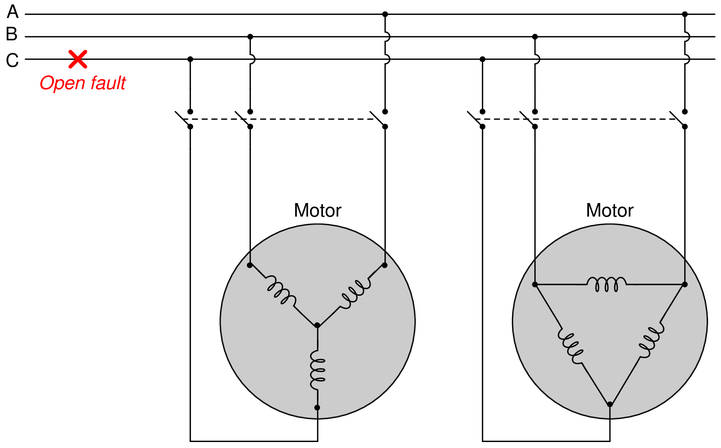Which of these two motor designs will fare better in the event of a phase loss such as the open fault in phase C shown above, and why?

Reveal answer

### Related Content

• Share

Published under the terms and conditions of the Creative Commons Attribution License

0 Comments